### Home > CALC > Chapter 12 > Lesson 12.1.5 > Problem12-58

12-58.
1. The graph below shows the graph of the derivative of f(x). Give approximate answers to the questions below and justify your responses. Homework Help ✎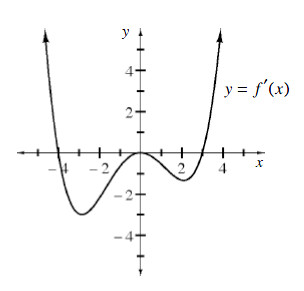1. a. For what values of x in the interval [−4, 4] does f attain a relative minimum?

2. At what value of x does a critical point that is neither a relative maximum nor a relative minimum exist?

3. For what values of x in the interval [−4, 4] is the graph of f concave up?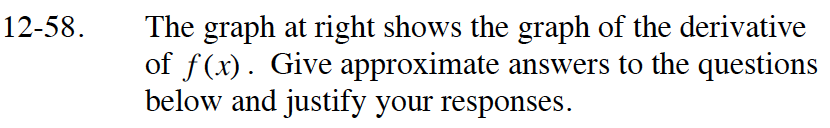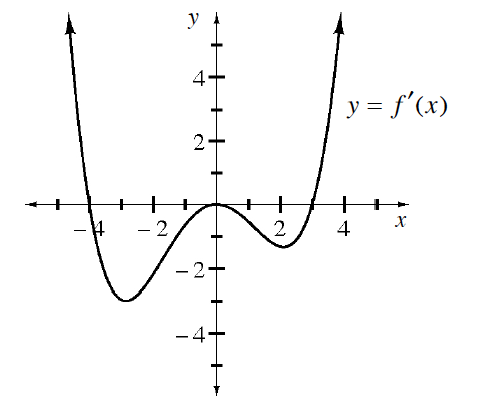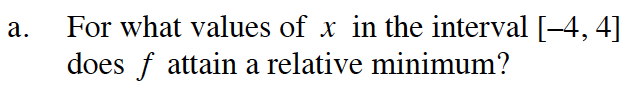This occurs when f′(x), or the slope function, changes from negative to positive.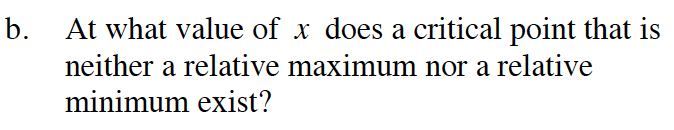This occurs when f′(x) = 0, but does not change from negative to positive.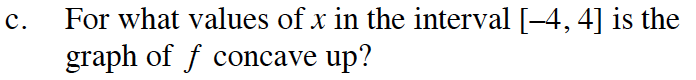This occurs when f′(x) > 0.